BiggerNumberGame

# ThreeFiveEight - Hot Game Show Riddler¶

This is an attempt at solving this ridder by the folks at 538.

This entire post is a Jupyter notebook, and it uses Python (Pandas and Numpy) to explore different aspects of the riddle.

### The Problem¶

Two players go on a hot new game show called â€œHigher Number Wins.â€ The two go into separate booths, and each presses a button, and a random number between zero and one appears on a screen. (At this point, neither knows the otherâ€™s number, but they do know the numbers are chosen from a standard uniform distribution.) They can choose to keep that first number, or to press the button again to discard the first number and get a second random number, which they must keep. Then, they come out of their booths and see the final number for each player on the wall. The lavish grand prize â€” a case full of gold bullion â€” is awarded to the player who kept the higher number. Which number is the optimal cutoff for players to discard their first number and choose another? Put another way, within which range should they choose to keep the first number, and within which range should they reject it and try their luck with a second number?

Note: If you have attempted this problem first, then you can follow along easily and see where the approaches vary from what you attempted.

### Solution Approaches¶

There are at least two good ways to approach this riddle. If you are a programmer and like to let the computer all the hard work, then a numerical simulation approach is the first thing that will occur to you. On the other hand, if you like to work out probabilities, then you prefer the analytical approach.

We will start with the Numerical Simulation approach, then also calculate the probabilities.

### Intuition¶

Before we even begin calculating, our intuition strongly suggests that a player would want to use 0.5 as the cutoff. But can we prove this? This post is an attempt to see if our initial guess was correct.

As it turns out, things are easier if we think of the events of this Game Show as a decision tree.

### Decision Tree¶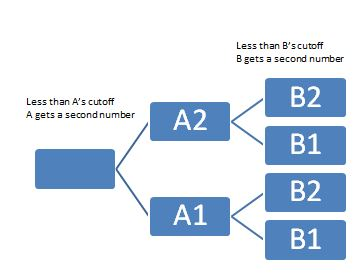## Method 1: The Numerical Simulation Approach¶

Let's say this game was played a million times. We can simulate that using Uniform random numbers. We can then count out the number of time each player won, under different scenarios. The scenarios in this experiment would be the different cutoffs that each player decides on.

In :
%matplotlib inline
import matplotlib
import matplotlib.pyplot as plt
import numpy as np
import pandas as pd
import sys, os

In :
millU = np.random.uniform(0,1,int(4e6)).reshape(1000000,4)
df = pd.DataFrame(data=millU, columns=['a1','a2','b1','b2'])

In :
df.shape

Out:
(1000000, 4)
In :
df.head()

Out:
a1 a2 b1 b2
0 0.678229 0.906019 0.140983 0.691082
1 0.242459 0.210721 0.816614 0.172865
2 0.473276 0.731607 0.710147 0.124943
3 0.289715 0.730348 0.575624 0.980886
4 0.300231 0.651216 0.122309 0.416013

### Compute "Prob of Player A winning" for different CUTOFF point choices for each player¶

Now that we have a ready-made million row dataframe at our disposal, we can try out as many experiments as we like.

For example, we could choose a cutoff for A, and one for B, and see how often player A ends up winning.

Let's try it out. Say Player A chooses to stand at 0.2. (Meaning that if player A gets above 0.2, they won't ask for different number.) Meanwhile, Player B chooses to go for a bigger cuttoff. That player chooses 0.7 as their cutoff. In this scenario, how often will player A win?

We can do that easily by creating a couple of new columns in our dataframe, and seeing which of those two final numbers is greater. We can then convert that number to a percentage, since we know we have a million rows.

In :
CUTOFF_A = 0.2 #The number above which A will not ask for another hit (second number)
CUTOFF_B = 0.7 #The number above which B will not ask for another hit (second number)

#Create two new columns
df['Final_A'] = (df['a1']<CUTOFF_A)*df['a2']+ (df['a1']>CUTOFF_A)*df['a1']
df['Final_B'] = (df['b1']>CUTOFF_B)*df['b1'] + (df['b1'] < CUTOFF_B) * df['b2']

#Finally, we create a column of True/False to denote who won
df['A_Won'] = (df['Final_A']>df['Final_B'])
prob_A_winning = (df['Final_A']>df['Final_B']).sum()/1e6
print prob_A_winning

0.460286


That seems to work. Now, we could just do this for many, many pairs of cutoffs. Which is what we are going to do next.

But before that, if makes sense to make the computation above into a Python function. Let's make it a function, so that we can call it repeatedly, giving it different A and B Cutoff values.

### Writing a Function to Simulate the Win Probability¶

In :
def prob_A_winning_simulation(CUTOFF_A, CUTOFF_B):
df['Final_A'] = (df['a1']<CUTOFF_A)*df['a2']+ (df['a1']>CUTOFF_A)*df['a1']
df['Final_B'] = (df['b1']>CUTOFF_B)*df['b1'] + (df['b1'] < CUTOFF_B) * df['b2']
df['A_Won'] = (df['Final_A']>df['Final_B'])
return (df['A_Won']).sum()/1e6


Let's test it to see if this function is working as we would expect.

In :
print prob_A_winning_simulation(0.2, 0.7) #does it match what we computed before?
print prob_A_winning_simulation(0.33, 0.75)
print prob_A_winning_simulation(0.1,0.1)

0.460286
0.499826
0.499114


### Running a Grid of Cutoffs¶

Now that we have a function ready, we can let the a and b cutoff's vary from 0 to 1, get the probability of A winning in each case, and store those. In this case, let's say that we take steps of 0.02, so there will be 50x50 = 2500 runs we make. So, the results list will have 2500 rows.

In :
results = []
step = 0.02
nparray = np.arange(0,1,step)
for sta in  nparray:
for stb in  nparray:
results.append((sta, stb, prob_A_winning_simulation(sta, stb)))

sim_results_df = pd.DataFrame(results, columns=['A','B', 'probA']) #cast the results in a pandas df

In :
#sim_results_df[sim_results_df['B']==0.5]


Logically, the next step is to examine our results data frame. We want to know the cutoffs for A which helped the most.

In :
rcParams['figure.figsize'] = 3, 3

fig = plt.figure()
data = sim_results_df[sim_results_df['B']==0.5]
data.plot(x='A', y='probA')
plt.suptitle('Win Probabilities over cutoff values, if opponent chose 0.5 as the cutoff')
plt.xlabel('cutoff for player')
plt.ylabel('probability of winning')
plt.xlim(0.48, 0.7)

Out:
(0.48, 0.7)
<matplotlib.figure.Figure at 0x33ac2198>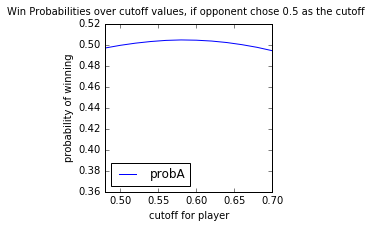### Plotting Functions¶

Next, let's create a couple of plotting functions (using MatPlotLib in Python) which we can call to create surface plots. For now, you can skip over these. (Refer to them if you are interested in recreating similar plots. I have used pcolor. In the winloss plot, I use only two colors. One to show cells where the probability of winning is above 0.5, the other to denote lower than 0.5. In the other surface plot, the color indicates the probability value. 1.0 is green, and 0.0 is red.

In :
def plot_winloss(rdf, step):
from pylab import rcParams
rcParams['figure.figsize'] = 8,8

nparray = np.arange(0,1,step)
width = len(nparray)
fig, ax = plt.subplots()
data = (rdf['probA']>=0.499999).values.reshape(width, width)
heatmap = ax.pcolor(data, cmap="autumn")

# put the major ticks at the middle of each cell
ax.set_xticks(np.arange(data.shape)+0.5, minor=False)
ax.set_yticks(np.arange(data.shape)+0.5, minor=False)

# want a more natural, table-like display
ax.invert_yaxis()
ax.xaxis.tick_top()

ax.set_xticklabels(nparray, minor=False)
labels = [item.get_text() for item in ax.get_xticklabels()]
for idx,l in enumerate(labels):
if (idx % 5):
labels[idx] = ""
ax.set_xticklabels(labels, minor=False)

ax.set_yticklabels(labels, minor=False)
plt.show()

def plot_win_surface(rdf, step):
from pylab import rcParams
rcParams['figure.figsize'] = 8,8

#step = 0.02
nparray = np.arange(0,1,step)
width = len(nparray)

fig, ax = plt.subplots()
data = rdf['probA'].values.reshape(width, width)
heatmap = ax.pcolor(data, cmap="RdYlGn")

# put the major ticks at the middle of each cell
ax.set_xticks(np.arange(data.shape)+0.5, minor=False)
ax.set_yticks(np.arange(data.shape)+0.5, minor=False)

# want a more natural, table-like display
ax.invert_yaxis()
ax.xaxis.tick_top()

ax.set_xticklabels(nparray, minor=False)
labels = [item.get_text() for item in ax.get_xticklabels()]
for idx,l in enumerate(labels):
if (idx % 5):
labels[idx] = ""
ax.set_xticklabels(labels, minor=False)

ax.set_yticklabels(nparray, minor=False)
plt.suptitle('Win Probabilities Heatmap')
plt.xlabel('cutoff chosen by opponent')
plt.ylabel('Cutoff chosen by Player')

plt.show()


Let's now plot our simulation results.

In :
step = 0.02
plot_win_surface(sim_results_df, step)
plot_winloss(sim_results_df, step)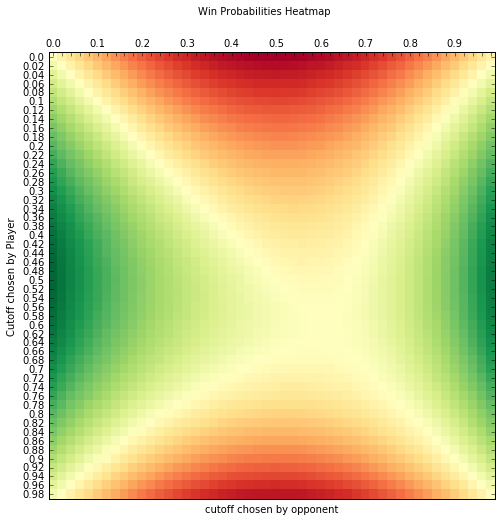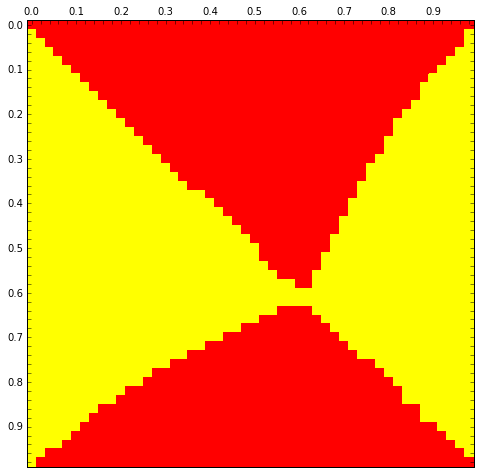In :
prob_A_winning(0.6,0.5)

Out:
0.50490000000000002

The numerical simulation is working. Let's also try calculating the probabilities.

## Method 2: Analytical Approach: Calculating the probability¶

### Developing some Intuition¶

We know that the probability of a number U(0,1) beating another number in the same range: U(0,1) is half. But what is the probability if the intervals are different? We can work that out...If a = 0.3 and b=0.7, this works out to be (1-0.7)/(1-0.3) * 0.5 = 3/14

In :
cola = np.random.uniform(0.3,1,int(1e6))
colb = np.random.uniform(0.7,1,int(1e6))
#data = pd.DataFrame({'a': cola,'b':colb})
sum(cola>colb)/1e6, 3/14.0

Out:
(0.214, 0.21428571428571427)

That matches. So now, we can work out the probability for each of the 4 cases in our decision tree.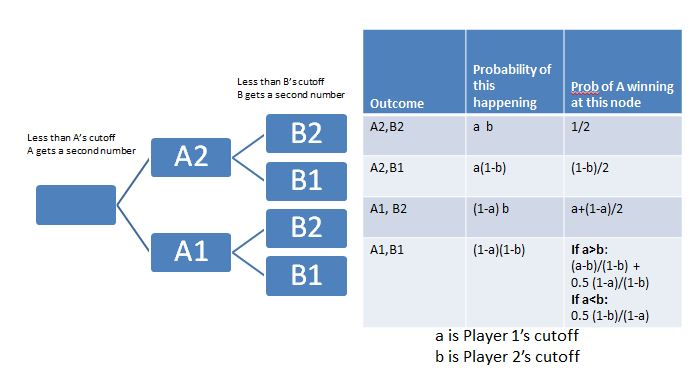In :
def analytical(a,b):
'''
Function returns the probability of Player A winning (getting a higher number)
if player A chose a as the cutoff, and the opponent chose b as the cutoff
'''
pwin = a*b *0.5
pwin += a*(1-b)*((1-b)/2)
pwin += (1-a)*b *(a+((1-a)/2))
ev = (1-a)*(1-b)
if a > b:
p = ((a-b)/(1-b)) + (0.5 * (1-a)/(1-b))
pwin += ev * p
else: #a is smaller. a---1 vs b--1
pwin += ev * ((1-b)/(1-a))*0.5
return pwin



Let's see how well the analytical approach matches the numerical simulation...

In :
acut = 0.6
bcut = 0.5
print analytical(acut, bcut), prob_A_winning(acut,bcut)

0.505 0.5049


That seems to match well. So looks like our analytical function is working. Now, let's just call it a bunch of times, with varying A and B cutoff values, and store the resulting probability in a results DataFrame (called 'rdf')

In :
aresults = []
step = 0.01
nparray = np.arange(0,1,step)
width = len(nparray)
for sta in  nparray:
for stb in  nparray:
aresults.append((sta, stb, analytical(sta, stb)))

rdf = pd.DataFrame(aresults, columns=['A','B', 'probA'])


Let's examine the results. We can try plotting different aspects of rdf

In :
step = 0.01
print rdf.shape
plot_win_surface(rdf, step)
plot_winloss(rdf, step)

(10000, 3)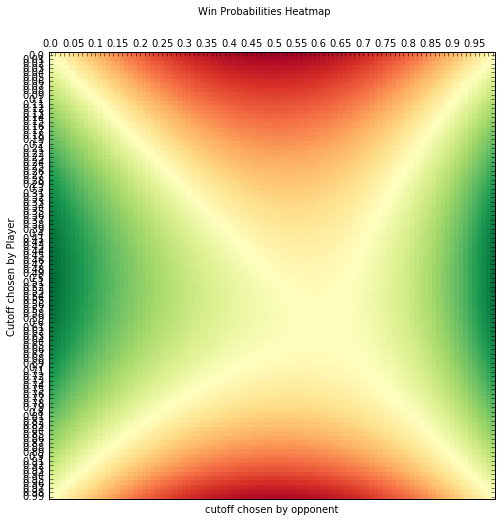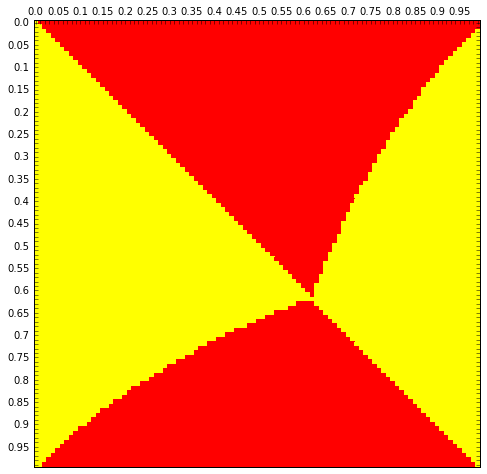In :
meanprob = []
for a in rdf.A.unique():
cond = rdf['A']==a
meanprob.append((a, rdf[cond]['probA'].mean()))

data = pd.DataFrame(meanprob, columns=["A", 'probA'])
data.plot(x='A', y='probA')

plt.suptitle('Mean Win Probabilities over all cutoff values for opponent')
plt.xlabel('cutoff for player')
plt.ylabel('probability of winning')

Out:
<matplotlib.text.Text at 0x4c834e48>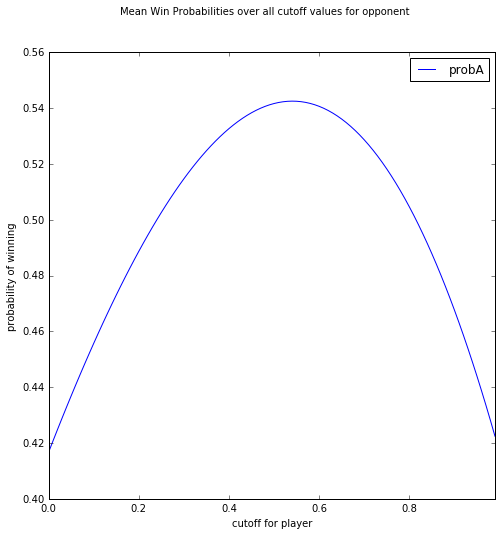In :
rcParams['figure.figsize'] = 7, 7

fig, ax = plt.subplots()

for temp in [x * 0.1 for x in range(0, 10)]:
data = rdf[rdf['B']==temp]
ax.plot(data['A'], data['probA'], label = "{0}".format(temp))

plt.suptitle('Win Probabilities over cutoff values')
#plt.axhline(y=0.5)
plt.axvline(x=0.61)

plt.xlim(0.4, 0.8)
plt.xlabel("A's cutoff")
plt.ylabel("Pr(A Winning)")
ax.legend()
plt.show()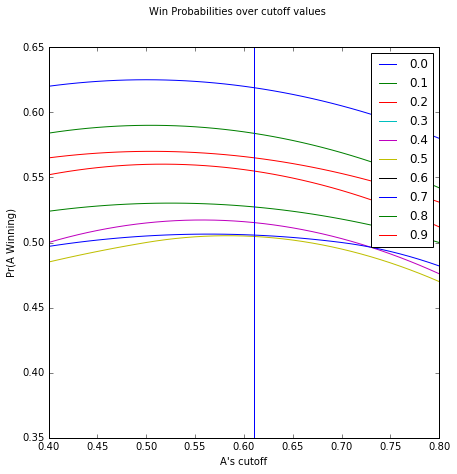From the plot above, we can see that if a player chooses a cutoff value of slightly above 0.6, they maximize their chance of winning the game show. (I.e., no matter what strategy the opponent chooses to adopt, the first player's win probability is above 0.5.)

In :
cond = rdf['A']==0.62
print rdf[cond]['probA'].min(), rdf[cond]['probA'].max()
#compared against:
cond = rdf['A']==0.50
print rdf[cond]['probA'].min(), rdf[cond]['probA'].max()

0.5 0.6178
0.4948 0.625


It looks like our intuition didn't serve us well in this case.

#### 1 comment:

1.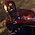The best Halo Multiplayer so far by a long shot. Campaign is sweet but it's not quite the best. I really recommend Halo 5, I haven't had this much fun with a game in a LONG time. 9.5/10
See more review: Jurassic World Evolution
Smoke and Sacrifice
Moonlighter